Importance: High ✭✭✭
 Author(s): Schrijver, Alexander Seymour, Paul D.
 Subject: Combinatorics » Matroid Theory
 Keywords: matroid sumset zero sum
 Posted by: mdevos on: June 10th, 2007

Letbe an (additive) abelian group, and for every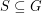let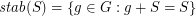.

Conjecture   Let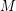be a matroid on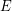, letbe a map, putand. ThenAlthough this conjecture may look a bit technical, it is in fact very natural, and important.

There is an interesting branch of combinatorial number theory which begins with the Cauchy-Davenport theorem, and M. Kneser's generelization of this theorem. We highlight these two theorems below. For a positive integer, we let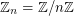.

Theorem  (Cauchy-Davenport)   Ifis prime andare nonempty, then.
Theorem  (Kneser)   Let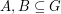be finite and nonempty, and let. Then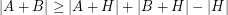.

In a somewhat underappreciated paper of Schrijver and Seymour, they find a generalization of the Cauchy-Davenport theorem to matroids. Namely, they prove the following.

Theorem  (Schrijver, Seymour)   Letbe a matroid on, letbe prime, and let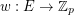be a map. Then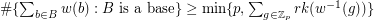.

The special case of this theorem when the underlying matroid is obtained from the free matroid on two elements by adding parallel edges is exactly the Cauchy-Davenport theorem. Further, their conjecture is precisely the common generalization of their theorem and Kneser's theorem.

DeVos, Goddyn, and Mohar have proved this conjecture in the special case when the underlying matroid is obtained from a uniform matroid by adding parallel elements, but apart from that, little is known.

## Bibliography

[C] A.L. Cauchy, Recherches sur les nombers, J. Ecole Polytechniques, 9 (1813), 99-123.

[D] H. Davenport, On the addition of residue classes, J. London Math. Soc., 10 (1935), 30-32.

[K] M. Kneser, Abschätzung der aymptotischen dichte von summenmengen, Math. Z. (1953) 459-484.

[N] M.B. Nathanson, Additive Number Theory, GTM 165, Springer, 1996.

*[SS] A. Schrijver and P.D. Seymour, Spanning trees of different weights. Polyhedral combinatorics, DIMACS Ser. Discrete Math. Theoret. Comp. Sci., 1, 281-288.

* indicates original appearance(s) of problem.